Drop a Query

# R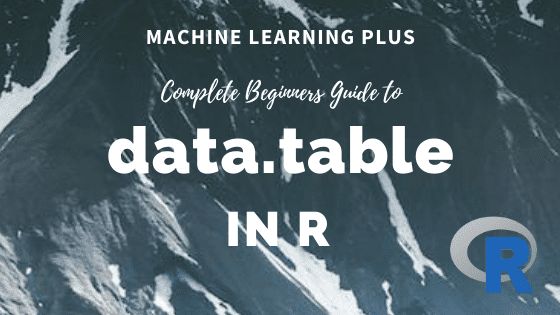## data.table in R – The Complete Beginners Guide

data.table is a package is used for working with tabular data in R. It provides the efficient data.table object which is a much improved version of the default data.frame. It is super fast and has intuitive and terse syntax. If you know R language and haven’t picked up the data.table package yet, then this tutorial …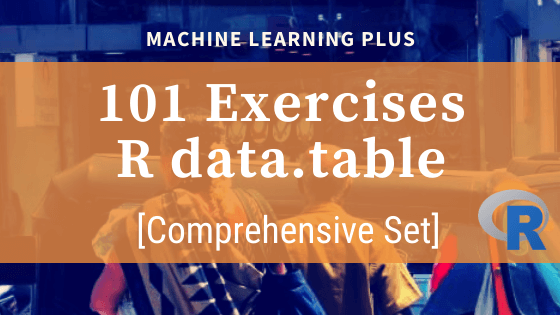## 101 R data.table Exercises

The data.table package in R is super fast when it comes to handling data. It has a syntax that reduces keystrokes while making R code easier to read. These set of exercises are designed to help you to oil your data brain through solving data manipulation exercises. Related post: 101 Python datatable Exercises (pydatatable) 101 …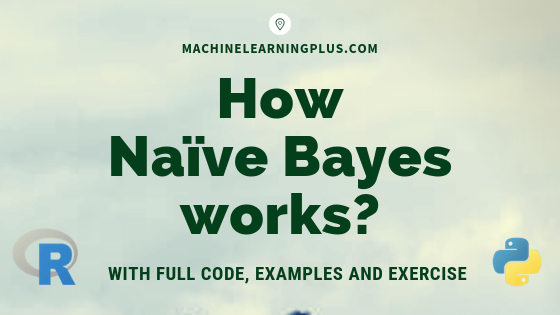## How Naive Bayes Algorithm Works? (with example and full code)

Naive Bayes is a probabilistic machine learning algorithm based on the Bayes Theorem, used in a wide variety of classification tasks. In this post, you will gain a clear and complete understanding of the Naive Bayes algorithm and all necessary concepts so that there is no room for doubts or gap in understanding. Contents 1. …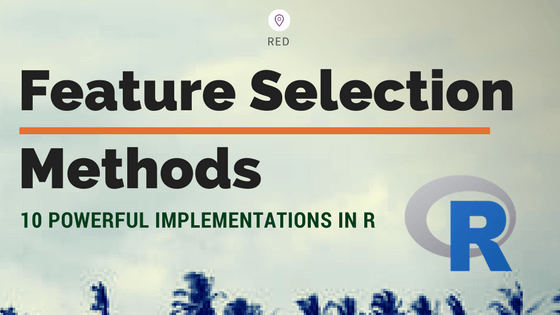## Feature Selection – Ten Effective Techniques with Examples

In machine learning, Feature selection is the process of choosing variables that are useful in predicting the response (Y). It is considered a good practice to identify which features are important when building predictive models. In this post, you will see how to implement 10 powerful feature selection approaches in R. Introduction 1. Boruta 2. …## Caret Package – A Practical Guide to Machine Learning in R

Caret Package is a comprehensive framework for building machine learning models in R. In this tutorial, I explain nearly all the core features of the caret package and walk you through the step-by-step process of building predictive models. Be it a decision tree or xgboost, caret helps to find the optimal model in the shortest …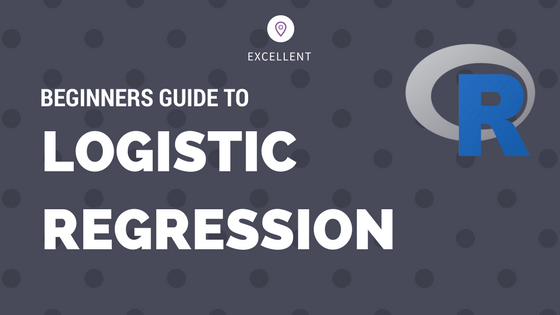## Logistic Regression – A Complete Tutorial With Examples in R

Logistic regression is a predictive modelling algorithm that is used when the Y variable is binary categorical. That is, it can take only two values like 1 or 0. The goal is to determine a mathematical equation that can be used to predict the probability of event 1. Once the equation is established, it can …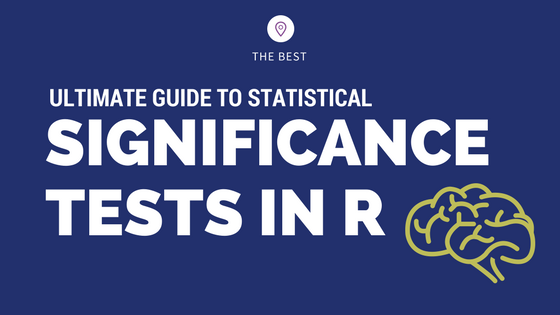## How to implement common statistical significance tests and find the p value?

How to implement and interpret the commonly used statistical significance tests in R? Understand the purpose, when to use and how to interpret the test results and the p value. Correlation Test and Introduction to p value One Sample t-Test Wilcoxon Signed Rank Test Two Sample t-Test and Wilcoxon Rank Sum Test Shapiro Test Kolmogorov …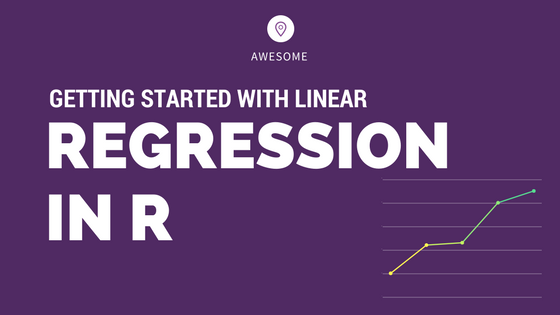## Complete Introduction to Linear Regression in R

We have covered the basic concepts about linear regression. Besides these, you need to understand that linear regression is based on certain underlying assumptions that must be taken care especially when working with multiple Xs. Once you are familiar with that, the advanced regression models will show you around the various special cases where a …Course Preview

## Machine Learning A-Z™: Hands-On Python & R In Data Science

### Free Sample Videos:#### Machine Learning A-Z™: Hands-On Python & R In Data Science#### Machine Learning A-Z™: Hands-On Python & R In Data Science#### Machine Learning A-Z™: Hands-On Python & R In Data Science#### Machine Learning A-Z™: Hands-On Python & R In Data Science